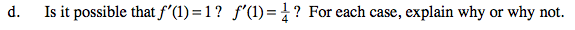### Home > CALC > Chapter Ch3 > Lesson 3.4.1 > Problem3-153

3-153.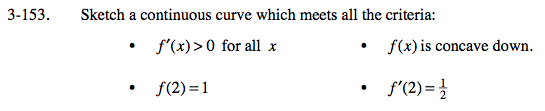f'(x) > 0 means f(x) is always increasing.

f(x) looks something like a square root graph (w/out the endpoint) or a logarithmic graph (w/out the asymotote).

If f(2) = 1, then the point (2,1) lies on the graph of the function.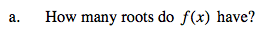Is it possible for a function that is always increasing (hint 1) AND always concave down (hint 2) to have no roots?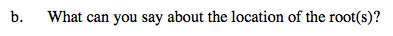On what domain could the root(s) not exist?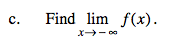Could there be a horizontal asymptote as x → −∞?